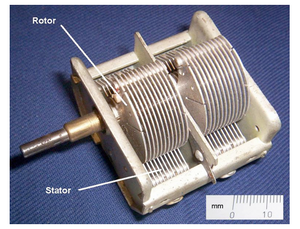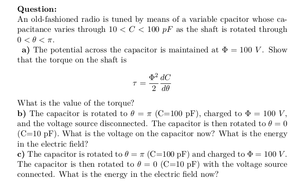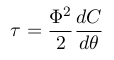Solved Problems in Physics, Physical Chemistry, and Computer Simulation Projects for College and Graduate Students.# Variable Capacitor in an Old-Fashioned Radio

Regular price
\$4.00
Sale price
\$4.00
Tax included.

Question:

An old-fashioned radio is tuned by means of a variable capacitor whose capacitance varies through 10 < C < 100 pF as the shaft is rotated through 0 < θ < π.
a) The potential across the capacitor is maintained at Φ = 100 V . Show that the torque on the shaft isWhat is the value of the torque?

b) The capacitor is rotated to θ = π (C=100 pF), charged to Φ = 100 V, and the voltage source disconnected. The capacitor is then rotated to θ = 0 (C=10 pF). What is the voltage on the capacitor now? What is the energy in the electric field?

c) The capacitor is rotated to θ = π (C=100 pF) and charged to Φ = 100 V . The capacitor is then rotated to θ = 0 (C=10 pF) with the voltage source connected. What is the energy in the electric field now?

By purchasing this product, you will get the step by step solution of the above problem in pdf format and the corresponding latex file where you can edit the solution. I am also available to help  you with any possible question you may have.